# Spatial and temporal point tracking in real hyperspectral images

## Abstract

In this article, we consider the problem of tracking a point target moving against a background of sky and clouds. The proposed solution consists of three stages: the first stage transforms the hyperspectral cubes into a two-dimensional (2D) temporal sequence using known point target detection acquisition methods; the second stage involves the temporal separation of the 2D sequence into sub-sequences and the usage of a variance filter (VF) to detect the presence of targets using the temporal profile of each pixel in its group, while suppressing clutter-specific influences. This stage creates a new sequence containing a target with a seemingly faster velocity; the third stage applies the Dynamic Programming Algorithm (DPA) that tracks moving targets with low SNR at around pixel velocity. The system is tested on both synthetic and real data.

## Introduction

In the intervening years, interest in hyperspectral sensing has increased dramatically, as evidenced by advances in sensing technology and planning for future hyperspectral missions, increased availability of hyperspectral data from airborne and space-based platforms, and development of methods for analyzing data and new applications .

This article addresses the problem of tracking a dim moving point target from a sequence of hyperspectral cubes. The resulting tracking algorithm will be applicable to many staring technologies such as those used in space surveillance and missile tracking applications. In these applications, the images consist of targets moving at sub-pixel velocities on a background consisting of evolving clutter and noise. The demand for a low false alarm rate on the one hand, and a high probability of detection on the other makes the tracking a challenging task. We posit that the use of hyperspectral images will be superior to current technologies using broadband IR images due to the ability of the hyperspectral image technique to simultaneously exploit two target-specific properties: the spectral target characteristics and the time-dependent target behavior.

The goal of this article is to describe a unique system for tracking dim point targets moving at sub-pixel velocities in a sequence of hyperspectral cubes or, simply put, in a hyperspectral movie. Our system uses algorithms from two different areas, target detection in hyperspectral imagery  and target tracking in IR sequences . Numerous works have addressed each of these problems separately, but to the best of our knowledge, to date no attempts have been made to combine the two fields.

We chose the most intuitive approach to tackle the problem, namely, divide and conquer; we separate the problem into three sub-problems and sequentially solve each one separately. Thus, we first transform each hyperspectral cube into a two-dimensional (2D) image using a hyperspectral target detection method. The next step involves a temporal separation of the movie (sequence of images) into sub-movies and the usage of a variance filter (VF)  algorithm. The filter detects the presence of targets from the temporal profile of each pixel, while suppressing clutter-specific influences. Finally, a track-before-detect (TBD) approach is implemented by a dynamic programming algorithm (DPA), to perform target detection in the time domain [1417, 19]. Performance metrics are defined for each step and are used in the analysis and optimization.

To evaluate the complete system, we need to obtain a hyperspectral movie. Since data of this kind are not yet available to us, an algorithm was developed for the creation of a hyperspectral movie, based on a real-world IR sequence and real-world signatures, including an implanted synthetic moving target, given by Varsano et al. .

## 1 System Architecture

The system performs target detection and tracking in three steps: a match target spectral filter, a sub-pixel velocity match filter (MF), and a TBD filter. This third step proves to be an effective algorithm for the tracking of moving targets with low signal-to-noise ratios (SNRs). The SNR is defined as: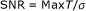(1)

where MaxT is the target's maximum peak amplitude and σ is the standard deviation.

The general system architecture is given in Figure 1.

Parts of this study have been published previously by our group: we will, therefore, refer extensively to those publications. Algorithms for target detection in single hyperspectral cubes are described in Raviv and Rotman , the details of the VF and of the generation of the hyperspectral movie are presented in Varsano et al. , and the DPA is described in Nichtern and Rotman . In this article, we present an overall integration of the system; in particular, the article analyzes the integration of the VF and the DPA and provides an overall evaluation of the system.

### Step 1: Transformation of the hyperspectral cube into a 2D image - the hyperspectral reduction algorithm

Three different reduction tests - spectral average, scalar product, and MF - were applied on each temporal hypercube individually. Each of these methods is characterized by a mathematical operator, which is calculated on each pixel. In every frame, a map of pixel scores is obtained (the result of the mathematical operator) and used to create the movie.

#### Test 1: spectral average

This test involves implementation of a simple spectral average of each pixel by: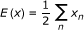(2)

where x denotes the pixel's spectrum, x n the spectral value of the nth band, and N the number of spectral bands.

#### Test 2: scalar product

Test 2 is a simple scalar product of the pixel's spectrum (after mean background subtraction) with the known target spectral signature: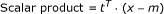(3)

where x is the pixel being examined, t is the known target signature, and m is the background estimation based on neighboring pixels.

#### Test 3: MF

In every frame, a map of pixel scores is obtained (the result of the mathematical operator) and used to create the movie. The article assumes the linear mixture model (LMM) of the background and a known target signature. The MF detector is given as follows: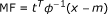(4)

where x is the pixel being examined, t is the known target signature, and m and Φ are the background and covariance matrix estimations, respectively.

The background subtraction procedure is done prior to applying the filter. The background estimation is performed on the closest neighbors that definitely do not contain the target; for example, if the target is known to be at most two pixels wide, the background is estimated from the 16 surrounding neighbors, as illustrated in Figure 2.

The MF test was run with different target factors (intensities). The target factor (intensity) can be controlled manually by the hyperspectral data creation algorithm, as an external parameter to the three tests mentioned previously. A higher target factor value, i.e., stronger intensity of the implanted target, poses less difficulty to the detection and tracking algorithm. Since the target implantation model is linear, it is directly proportional to the target factor (intensity of implantation).

Overall, the input to the first stage is a hyperspectral cube; the output of the first stage is a 2D image obtained from the hypercube. Details of the signal processing algorithm using a hyperspectral MF can be found in Raviv et al. .

### Step 2. Temporal separation of the 2D sequence: the temporal processing algorithm

Buffering a number of 2D images acquired in step one is needed to obtain a sequence that is sufficiently long to perform temporal processing with the VF . The input for the temporal processing algorithm is the temporal profile of a pixel. Figure 3 defines our terminology at this stage.

The temporal processing algorithm starts with a temporal separation of each temporal profile into sections; each section should roughly cover the time it takes for the target to enter and leave the pixel. By compressing each section into a single picture, the original amount of sequence images will now be condensed into a smaller sequence of images with the target moving at pixel velocities (at least one pixel per frame). For example, the profile in Figure 4 (top) is an input to the temporal separation which increases the velocity of the target to at least one pixel per frame, as shown in Figure 4 (bottom). The number of sub-profiles is defined by:(5)

where N is the number of profile frames, G0 is the overlap between each of the sub-profiles, and G is the length of the sub-profile.

Following temporal separation, the temporal processing algorithm is applied. The temporal processing algorithm is based on a comparison of the sub-profile overall linear background estimation (defined as DC) to the single highest fluctuation within the sub-profile. The overall linear background estimation (DC) fit, is done using a wider temporal window of samples to achieve best background estimation. The background estimation is performed by calculating a linear fit by means of least squares estimation (LSE) . The fluctuation or short-term variance estimation is performed on a short temporal window of samples (susceptible to temporal variations, i.e., the target entering/leaving pixel), after removing the estimated baseline background. The algorithm is presented in the following two steps, although, in practice, the processing can be performed in real time using a finite size buffer.

#### Background estimation using a linear fit model

The background can be regarded as the DC level of the temporal profile: the DC level is constant for noise-dominated temporal profiles but varies with time when clutter is present. The DC is estimated in a piecewise fashion using a long-term sliding window and performing the estimation on each set of samples separately. The number of samples for each long-term window is denoted by M. The following linear model is used for estimating the DC; for the sake of simplicity, the description of the estimation is applied to a single window: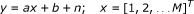(6)

where n is the noise, a and b are the coefficients that must be estimated, M is the number of samples for each long-term window, and y is the DC signal. The goal of this step is to estimate the long-term DC baseline using a least-squares fit to the linear model represented by a coefficients vector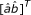.

Equation 6 can be rewritten as follows: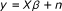(7)

where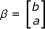and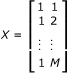Using LSE, the following equation is obtained for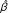: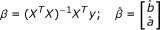(8)

The estimated DC of a single window thus becomes: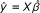(9)

The estimated DC of the complete signal is obtained after performing the above calculations for each window separately. Figure 5 shows two synthetic temporal profiles (one with the target implanted and the other with identical noise but without a target) and their estimated DC signals.

The estimated DC is based on the entire temporal profile. The sub-profile DC estimation is chosen by the relative location within complete temporal pixel profile. Figure 6 shows the DC estimation on a signal with a target and the same signal without a target from the point of view of the sub-profiles separations.

#### Short-term variance estimation

The short-term variance calculation is performed after subtracting the estimated long-term DC from each sub-profile. The complete DC signal obtained in the previous step is denoted by DC j , where j denotes the index of sub-profile, and the number of sub-profiles is defined in Equation 5. DC j is subtracted from the temporal sub-profile P j :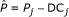(10)

The variance estimation is calculated by using a sliding short-term window and performing variance estimation on each set of samples separately. L denotes the number of samples in each short-term window. The short-term variance of each window is estimated as follows: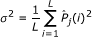(11)

For a window size of L samples, an overlap of L o samples, and a sub-temporal profile of G samples, the number of windows W is given by: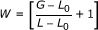(12)

Finally, the maximum variance of a given temporal profile is given by: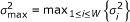(13)

where σ i 2 is the estimated variance of the ith window.

An example of the variance response to the presence of a target is shown in Figure 7. It is assumed that the presence of a target will lead to an increase in the short-term variance. The DC subtraction has a clutter suppression effect, since the long-term DC tracks the influence of clutter on the temporal profile. The graphs of sub-profiles 4 and 5 were scaled to the range of the pixel's values in the profile. The scaling was done with the aim of showing the range of the variance estimation values.

Finally, a likelihood-ratio-based metric is used to evaluate the final score of each sub-temporal profile. The likelihood ratio in this case is given by: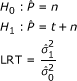(14)

whereis the zero-mean temporal profile, n is noise, and t is the target signal.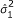is the estimated variance when assuming a target is present;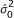is the variance estimated assuming the absence of a target.

In our model,variances are estimated as follows: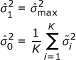(15)

where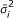for 1 ≤ iK denotes the K minimal variance values of each temporal profile. The value of K is chosen to be smaller than W, so as not to include values that might be caused by the presence of a target.

In this case, the final score of each sub-profile is given by: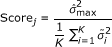(16)

The performance of the algorithm depends on a wise choice of parameters, i.e., the sizes of the short-term and long-term windows and the length of the sub-profile. The long-term window size serves as the baseline for DC estimation. Since the pixel might be affected by clutter, the baseline DC is not constant. It is assumed that the presence of clutter will cause a monotonic rise or fall pattern in the values of the pixel's temporal profile at least during the duration of the long-term window. Thus, the long-term window should be long enough to facilitate accurate estimation of background, on the one hand, and short enough to enable the influence of clutter to be tracked, on the other hand. Thus, the long-term window should be minimally longer than the target base width to avoid suppressing it . The short-term window is used for variance estimation. It should be matched (or reduced) to the target width (which depends on the target velocity). If the short-term window is significantly longer than the target width, the change in variance caused by the target will be reduced. The sub-profile length matches a pixel target velocity; it should be matched to the target temporal width. The importance of these two window sizes and the overall window parameters will be discussed in the experimental section of the article. We note that the temporal algorithm presented here does not assume a particular target shape and width. It does, however, assume a maximum temporal size of the target, (affecting the target temporal profile), and a positive adding of the target intensity to the background.

To determine the optimal set of window sizes on a real data sequence, the algorithm was run with various sets of parameters.

### Dynamic Programming Algorithm

The algorithm is implemented using the following assumptions :

1. 1.

The target size is one pixel or less.

2. 2.

Only one target exists in each spatial block.

3. 3.

The target may move in any possible direction.

4. 4.

Target velocity is within 0-2 pixels per frame (ppf).

5. 5.

Images are too noisy to allow detection of a threshold on a single frame.

6. 6.

Jitter of up to 0.5 ppf is allowed only in the horizontal and vertical directions and is uniformly distributed.

Since the target velocity is within the range of 0-2 ppf with a possible jitter of 0.5 ppf, the pixel can move up to 2.5 ppf in the horizontal and vertical directions; hence, a valid area from which a pixel might origin from in the previous frame is a 7 × 7 pixel area (matrix). Such a search area can be resized according to different velocity ranges and jitter values. The search area will define the probability matrices that contain the probabilities of pixels in the previous frames being the origin of the pixel in the current frame. To take into account unreasonable changes of direction, penalty matrices are introduced with the aim of building probability matrices for the different possible directions of movement. These matrices give high probabilities to pixels in the estimated direction and decreasing probabilities (punishment) as the direction varies from the estimated direction.

## 2 System Evaluation

### Evaluation of the temporal algorithm on synthetic data

#### Creation of synthetic IR frames

To evaluate the performance of the spatial and temporal tracking algorithms, synthetic temporal profiles that simulate different types of clutter and background behavior were created. A target signal was implanted into these background signals to simulate a target traversing both clutter and noise-dominated scenes. On the basis of the study of Silverman et al.  showing that the temporal noise is closely matched to white Gaussian noise, we used white Gaussian noise at various SNRs to test the temporal algorithm.

Figure 8 shows the different types of signal used to test the algorithm. The type 1 signal shown in Figure 8 simulated relatively fast and small clutter formation passing through a pixel. Signals of types 2, 3, and 4 simulated, respectively, slowly entering clutter, symmetrical slowly exiting clutter, and a noise-dominated scene in which the base timeline is constant. The type 5 signal served as reference signal, i.e., the best-case scenario, which comprises a constant zero-mean base line.

Target temporal profiles were characterized by a rapid rise and fall pattern. This behavior may be modeled by a half sine or triangular shape, as shown in Figure 9.

The base width of the target corresponds to the target velocity. The simulations showed that there were no significant performance differences between the sine and the triangular shaped targets.

Figure 10 shows the various background models with the sine shape implanted at an SNR of 4.

#### Examination of the temporal algorithm on synthetic data

This section demonstrates the algorithm's operation on the synthetic data described in the previous section. The following parameters affect the algorithm's performance:

1. 1.

The background type.

2. 2.

The SNR, which is a function of the noise variance and the target's amplitude (factor). SNR is a function of MaxT - the target's peak amplitude.

3. 3.

Parameters of the windowing procedure:

4. a.

the window size to estimate the background baseline DC: the grouping spatial window size to convert sub-pixel target velocity to the pixel target velocity in the frame (as an input to the DPA)

5. b.

the size of the short-term variance windows for each sample and for each grouping

6. c.

the step size of each window (overlapping).

The dependence of the performance of the algorithm on these factors is described below.

##### Background type

The factor most influenced by the background type is the DC estimation capability of the algorithm. It is expected that DC estimation will be easiest for signals having a constant DC level (signals of types 4 and 5) and for signals having a slowly changing DC (signals of types 2 and 3), since the linear regression is capable of estimating parameters of the linear model. Type 1 signals are the most problematic, since the DC of such signals does not have an overall fit with a linear model, but depends on piecewise matching of the DC to windows sizes, as explained below.

Figures 11, 12, 13, 14, 15, 16, 17, 18, 19, and 20 illustrate the algorithm's operation on the various signal types, with and without an implemented target. In each case, the DC signal and the estimated variance values (calculated after subtracting the estimated DC from the signal) are also plotted. The simulations were run for a DC window of 20 samples, a DC overlap of 50%, a sub-temporal profile of 15 samples, an overlap between sub-profiles of five samples, and an SNR of 4. The target width was 10 samples.

As can been seen in Figure 11, the increase in the variance of sub-profiles 2, 8, and 9 may be attributed to the imprecise DC estimation of the background. This case simulates a cloud entering and exiting. Nevertheless, the variance score of the target sub-profiles 5 and 6 is still much higher than that of sub-profiles 2, 8, and 9. The variance of the other sub-profiles 1, 3, 4, and 7 is close to zero.

The DC estimation for signals of types 2 and 3 is precise, since the signal fits a linear model. The variance increases significantly when the target passes through the pixel and is close to zero at other times.

Figures 14 and 15 shows a similar behavior for signals of different DC levels. As expected, the variance of each of signal (types 4 and 5) is the same.

Figures 16, 17, 18, 19, and 20 show the results of temporal processing (variance estimation) for the different signal types, for the cases where no target is present. Not all the sub-profiles are shown, since the values of the exhibited variance values are around zero.

From Figure 16 it can be seen that, by analogy with Figure 11, the variance increases for sub-profiles where the cloud enters and exits into/from frame. Such cases, i.e., with no target, may cause false alarms. Figures 17, 18, 19, and 20 show that the temporal processing score is close to zero for such signals in the absence of a target.

A simple means of performance evaluation is given by the ratio of the score obtained from the synthetic signal that contains a target to the same signal without a target, i.e., the target/noise (T/N) ratio. Table 1 shows the T/N ratio for the different signal types obtained by averaging 500 runs. It is important to note that the T/N ratio is not an effective metric to evaluate the algorithm, as will be shown later.

As expected, the performance of the type 1 signal is the worst among the different signals. Signals of types 2-5 all have similar good performance. If we compare our sub-temporal processing algorithm with a temporal processing algorithm as described in , it can be clearly seen (Table 1, 2nd column) that the performance improves by a factor of at least 2 for signals of types 2-5, but not for the type 1 signal, for which the performance improvement is insignificant.

##### Signal-to-noise ratio

As in many other applications, the SNR has a significant influence on the performance of the temporal algorithm. As the SNR increases, the algorithm's performance is expected to improve, since both the DC and variance estimation will be more accurate, as is shown in Figure 21.

The algorithm responds similarly for signals of types 2-5, in agreement with expectations, i.e., the performance improves as the SNR increases. The performance of the algorithm for the type 1 signal behaves differently; first it increases with the SNR, but at a slower rate than for signals of types 2-5, and it then decreases as a result of an inaccurate DC estimation, as will be detailed later. Since the DC of this signal does not fit a linear model and the estimation must therefore be performed in piecewise fashion, the size and the position of the windows used to perform the estimation act as a limiting factor to the performance.

##### Window size

Both the window size for the baseline DC estimation and the window size for the short-term variance for the sub-profile have a marked impact on the performance of the algorithm. It is expected that large window sizes for baseline DC estimation would improve the DC estimation in cases where the background changes monotonically (as for signals of types 2-4). Too large a DC estimation window size might, in some cases, lead to inaccurate tracking of the clutter form and cause high false alarm rates (e.g., as for type 1 signals). Thus, for background profiles, the optimal window size is determined by the background type, i.e., for a noise-dominated background or backgrounds containing monotonically changing clutter, larger window sizes are preferred; for backgrounds characterized by rapidly changing clutter, shorter windows are preferred. For target temporal profiles, the larger the DC window, the higher the profile score, since the presence of the target peak will have a smaller influence on the DC estimation. Obviously, an estimated DC that tracks the target form is highly undesirable since it leads to target suppression. Thus, in terms of the overall algorithm performance, the optimal DC window is the one that is small enough to closely track background changes but is large enough not to track the target peak.

The sub-temporal profile length should be matched to the target sub-pixel velocity, which is expressed as the base width of the peak of the target profile and the sub-pixel velocity, although there is no acute need for an exact match. The short-term variance window size for the sub-profile should be matched to the target rise time, although once again there is no acute need for an exact match. A sub-profile length that is larger than the target width will disable the ability to track/detect a target with a sub-pixel velocity. Alternatively, a sub-profile length that is smaller than the target width will allow too few samples for the sub-profile.

A short time variance window that is larger than the target rise time will result in a lower score for the target profile, since the variance calculated on each window is normalized by the window's length. Thus, for the target profile, the optimal variance window size is expected to be less than or equal to the sub-profile length. In fact, the shorter the window, the higher the score of the target profile. On the other hand, a short variance window is more sensitive to random noise spikes in a temporal profile dominated by noise. Therefore, the optimal variance window size for noise-dominated profiles should be as large as possible so as to diminish the effect of the noise spikes. The optimal variance window for the overall algorithm's performance is the one offering the best compromise between the need to enhance the target profile score (i.e., as short as possible) and the need to suppress the short-term noise fluctuations (i.e., as long as possible).

Another factor which will impact performance is the overlap window between the sub-profiles. The overlap window should allow for the compensation of low sub-pixel velocity that derives from a small sub-profile length. The overlap window results in the creation of more sub-profiles, as defined in Equation 5, since the greater number of sub-profiles aids to achieve a more accurate tracking estimation.

### Evaluation of the temporal processing algorithm on real data

#### Real IR sequences

Real-world IR image sequences taken from Silverman et al.  were used for evaluating the temporal algorithm. The movies comprised 95 or 100 12-bit IR frames. The sequences contain raw data of unresolved targets flying from Boston Logan Airport in Massachusetts, USA. In the available dataset, there are five scenes containing various types of clutter and sky as well as various targets moving at different velocities.

Figure 22 shows a single frame (frame 50) of each of the IR sequences examined in this study. Table 2 summarizes the number and the nature of the targets for each IR sequence and the background type of scene.

#### Evaluation metrics

Silverman et al.  suggested several performance metrics for the evaluation of temporal algorithms. A derived version of the metric was defined. Each frame in the sequence was divided into H × N blocks (30 × 30 were used), and the algorithm was run over nine blocks, i.e., the target block (TB) and its eight adjacent non-target blocks (NTB). The SNRs of the TB and its eight adjacent NTBs were calculated. Thereafter, the algorithm score was calculated on the basis of the resulting S NRs.

The block SNR is given as: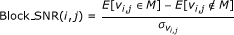(17)

where v i, j is the set of pixels belonging to the (i, j)th block, M is a set containing the five pixels with the highest gray level in that block, σ is the standard deviation of the block pixels. E[v i, j M] is the expected value of the highest pixels (target) and the E[v i, j M] is the expected value of the rest of the pixels (background).

The algorithm score is given as: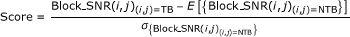(18)

The block formula performs a subtraction between the expectation value of the highest pixels (target) and the expectation value of the rest of the pixels (background), divided by the standard deviation of block pixels. Since the probability matrices of the DPA introduce the influence of target pixels on adjacent pixels, these influenced pixels might accumulate higher values than unaffected pixels (background), and can be regarded as target pixels. This might lower the expectation value of the target, but will also lower the standard deviation of the background, since these high pixels are higher than the statistics of the background.

The final grade of the algorithm serves as a tool for comparison between the suggested temporal processing algorithm and other temporal processing algorithms that deal with the same problems. The grade is a reflection of the difference between the score of the block containing the target and the expected values of the rest of the blocks in the image, normalized by their standard deviations.

#### Real data results

The real-world IR image sequences described in the previous section were used to evaluate the temporal tracking algorithm. The algorithm's output images are given in Figure 23, together with a representative frame from each sequence. The sequences were chosen so as to comprise both clutter- and noise-dominated scenes. The parameters of each simulation were chosen to be the optimal set, as will be explained in the following section. The target tracks are seen as bright short stripes, and in some cases, clutter leakage is also evident.

Figure 24 provides a closer view of the block containing the target of each output image together with a representative target temporal profile. Visual evaluation of the images presented in Figure 24 suggests that in terms of enhancement of the target pixels, the best results were obtained for the sequences J2A and NPA, since the target trace is stronger relative to the background. The M21F sequence, for example, has more noise in the background, although the target pixels are clearly visible. The metric defined for assessing the overall performance of the algorithm, which is given in Equation 17, takes into consideration not only the target enhancement but also the ability of the algorithm to suppress the background. This is achieved by grading each block with a score that evaluates the difference between the maximal 5 values of the block and the block average values, normalized by the standard deviation. The goal is, of course, to obtain TBs with high scores and background blocks with low scores.

The purpose of the final grading of the algorithm, defined in Equation 18 is to evaluate the separation between the TB(s) and the background blocks. The grading provides an evaluation of the difference between the TB score (and if there is more than one target, the mean of the TBs) and the mean of the background blocks, normalized by the standard deviation of the background. The final grade obtained for each sequence is given in Table 3. The table also shows the grade of the two temporal processing algorithms presented in Varsano et al.  - the VF and the NF and the temporal processing algorithm described in .

The variety of the target scores for the different sequences can be understood by examining the amplitude of the maximum target peak relative to the profiles average values.

A comparison of our and Varsano et al.  methods of temporal processing shows that scores that are given by the algorithm of Varsano et al.  are higher (except for NPA) than ours. The difference in the scores may be attributed to the differences in the metrics and TP calculation, i.e., the difference in the metric calculation is due to the fact that we have more score frames and hence we calculate their average, as described at Figure 25 and 25 in Table 1; the temporal processing method used gives us a better target to noise ratio.

The sequences in NPA differ from those in NA23: although the target in NPA seems weaker, its block score is higher than that of NA23 as a result of the strong clutter in the NA23 scene as shown at Table 4. As stated in the following section, the window size parameters are not optimized in terms of both the TB and the background. Thus, choosing an inappropriate window size will lower the TB score. Therefore, although the target in NA23 is stronger than that in NPA, the TB score obtained after the temporal processing is lower. Finally, the lowest target score is that for the M21F sequence. The target here is quite weak, and the scene is noise dominated, hence the low target amplitude and the low TB score. The algorithm score for this sequence was the lowest among the scores of all the sequences.

#### Optimal window size

Choosing the appropriate set of parameters for the temporal algorithm is crucial for the detection capabilities of the system. The dependence of the algorithm on the window size was evaluated on real data, and the optimal set of parameters was obtained for each IR sequence.

The expected optimal window sizes depend on both the shape of the target's temporal profile, mainly on the target's peak width, which is inversely proportional to the target's velocity, and on the background scene, i.e., on the presence of clouds and their size and velocity, as stated in section "Window size".

To determine the optimal set of window sizes on a real data sequence, the algorithm was run on the sequence with various sets of parameters. The set that yielded the highest algorithm grade, defined in Equation 18, was chosen for the evaluation.

Figure 26 shows the results of the simulation on the IR sequence NPA. The results show that the highest algorithm score was obtained for a group width of size 14 samples, an overlap of size 7 samples and a variance window of size 6 samples with a linear (DC) window of size 50 samples. The final algorithm grade provides an evaluation of the difference between the target's block score (if there is more than one target, the relevant scores are averaged) and the mean background score, normalized by the standard deviation of the background blocks. Thus, the optimal window set will tend to maximize the target score while minimizing the background and standard deviation scores. Table 5 summarizes the optimal window sets for three IR sequences.

### Evaluation of the complete system on real data

The hyperspectral movie is created as described in Varsano et al. . The movie consists of a sequence of 30 × 30 × 96 cubes (width × height × bands). A synthetic target is implanted into the sequence. The target is sine-shaped, 2 × 2 pixels wide, and has a horizontal and vertical velocity of 0.1 ppf. White Gaussian noise is added to each spectral signature and the noise variance is set to be [0.1 × Max(signature)]2.

The movie then constitutes the input into our system. The MF with the estimated covariance matrix is applied for the first-stage processing of each hypercube, and the temporal processing algorithm described in section "System architecture" is used for the target detection in the second stage. The output of the second stage constitutes the input into the DPA. The DPA allows us to track the target from pixel to pixel; an updated summation score for each pixel is kept for each pixel, based on its similarity to expected target behavior. Penalties are introduced to lower the score of pixels apparently acting in a non-physical manner. In the final stage, the last processed frame is taken and the highest pixel is declared as a "target" and its track is found. In this section, we will define three tests to evaluate the effect of each stage of the algorithm.

#### Metric definition

The performance metric, Equations 17 and 18, was used to evaluate various stages of the analysis. The three tests based on this metric were applied. Test 3 uses a MF for the cube collapsing. The resulting cubes are then evaluated as described in Equations 17 and 18; the cube with the highest score will be evaluated as representative of the efficacy of using the MF alone.

Test 4 (full system test) uses a MF detector as the input of the temporal processing velocity filter. Test 5 (full system test) adds to Test 4 the DPA. These tests were created to evaluate the effect of the IR tracking algorithms on the overall score of the hyperspectral tracking system.

The MF and the temporal processing algorithm create images with pixel scores according to their likelihood of being a target, whereas the DPA accumulates the scores of pixels according to the probability of the path going through them to be the target's path.

## Discussion of the results obtained on real data

Here, we present the results of applying the complete system algorithm on a hyperspectral movie based on blocks from the real IR sequence NA23. The algorithm was run on TBs and their eight surrounding background blocks. The blocks were chosen to represent three different scenes, which could roughly be categorized as "clear sky" (containing only sky), "weak clutter" (containing partial weak clutter (with low to medium IR amplitude)) and "strong clutter" (containing partial clutter with high IR amplitudes). The parameters of the simulation are summarized in Table 6.

Tables 7, 8, 9, 10, and 11 present the results for hypercubes of three different backgrounds, based on blocks from the real IR sequence NA23. Figure 27 shows a single frame from the sequence, divided into labeled blocks.

Comparison of Tables 9 and 10 shows that scores for Test 4 were lower than those for Test 3. This difference may be attributed to differences in the calculation of the metrics and the temporal processing method, i.e., the difference in the metric calculation due to the fact that we have more score frames and hence calculate their avarage (Figure 25); and as described in Table 1, the temporal processing method used gives us a better target to noise ratio.

Previous research  has shown that Test 1 allows a rough assessment of the relative amplitude of the target pixels vis-à-vis their background. The low values of the results indicate that the implantation of the target and taking the maximal score without any processing is not sufficient for target detection; in other words, the implantation method does not allow an "easy" detection. The highest values of Test 1 were obtained for the weak clutter scenes, which is reasonable since the implantation method is additive and in weak clutter surroundings, the amplitudes are obviously higher than those in clear sky scenes. A comparison of Tests 1 and 2 allowed us to estimate the improvement conferred by using primitive hyperspectral processing, i.e., simply taking the average of all the bands. Although this method led to an improvement in the sky and weak clutter scenes, it had negative impact on strong clutter scenes, a finding that indicates that simply averaging the bands is disastrous for certain sets of spectral signatures and cannot itself be used as a detection method. Thus, the discussion will focus on the use of "smart" hyperspectral processing (Test 3), hyperspectral processing and temporal processing (Test 4), and hyperspectral processing, temporal processing and the DPA (Test 5). The results of Varsano et al.  have shown that there is an obvious advantage of using both hyperspectral detection (MF) and temporal processing (Test 4 vs. Tests 1-3).

When the target is implanted in clear sky scenes, the use of temporal processing significantly improves the performance vis-à-vis hyperspectral detection alone. In most cases, the use of the MF compared to simple averaging was clearly advantageous, with the exception of block 31, for which the performance was similar for the two techniques. This similarity may be attributed to the relative "easiness" of detection in this kind of scene and the fact that the high level of noise might confer a disadvantage on the MF but an advantage on the averaging filter. When weak clutter was present, temporal processing combined with the MF detector was always better than hyperspectral detection alone.

A parameter known as the target factor (MaxT) has been used to describe linearly the power in the target signature. To define the boundaries of the full system, it is necessary to define a valid range for the target factor. Preliminary runs for target factor s of 1000, 500, 100, and 10 showed that target factor s of ≥ 100 are "too easy" to use a detection algorithm, whereas a target factor of 10 is "too hard" for the full system to detect and track a target. Therefore, trial runs were performed with the following values of the target factor: 20-80 with steps of 20. Figures 28 and 29 show the results for block 38 for target factors of 100 and 20, respectively, for various stages.

## 3 Conclusions

In this study, a complete system for the tracking of dim point targets moving at sub-pixel velocities in a sequence of hyperspectral cubes or, simply put, a hyperspectral movie was presented. Our research incorporates algorithms from two different areas, target detection in hyperspectral imagery and target tracking in IR sequences.

Performance metrics are defined for each step and are used in the analysis and optimization; a comparison is made to previous work in this area.

## Abbreviations

DPA:

dynamic programming algorithm

LSE:

least squares estimation

LMM:

linear mixture model

MF:

match filter

NTB:

non-target block

SNRs:

signal-to-noise ratios

TB:

target block

TBD:

track-before-detect

2D:

two-dimensional

VF:

variance filter.

## References

1. 1.

Chanussot J, Crawford MM, Kuo BC: Foreword to the special issue on hyperspectral image and signal processing. IEEE Trans Geosci Remote Sens 2010.,48(11):

2. 2.

Shaw G, Manolakis D: Signal processing for hyperspectral image exploitation. IEEE Signal Process Mag 2002, 19: 12-16.

3. 3.

Landgrebe D: Hyperspectral image data analysis as a high dimensional signal processing problem. IEEE Signal Process Mag 2002, 19: 17-28.

4. 4.

Shaw G, Burke H: Spectral imaging for remote sensing. Lincoln Lab J 2003,14(1):3-27.

5. 5.

Caefer CE, Rotman SR, Silverman J, Yip PW: Algorithms for point target detection in hyperspectral imagery. Proc SPIE 2002, 4816: 242-257.

6. 6.

Manolakis D, Shaw G: Detection algorithms for hyperspectral imaging applications. IEEE Signal Process Mag 2002, 19: 29-43. 10.1109/79.974724

7. 7.

Keshava N, Mustard JF: Spectral unmixing. IEEE Signal Process Mag 2002, 19: 44-57. 10.1109/79.974727

8. 8.

Stein DWJ, Beaven SG, Hoff LE, Winter EM, Schaum AP, Stocker AD: Anomaly detection from hyperspectral imagery. IEEE Signal Process Mag 2002, 19: 58-69. 10.1109/79.974730

9. 9.

Duran O, Petrou M: A time-efficient method for anomaly detection in hyperspectral images. IEEE Trans Geosci Remote Sens 2007, 45: 3894-3904.

10. 10.

Caefer CE, Mooney JM, Silverman J: Point target detection in consecutive frame staring IR imagery with evolving cloud clutter. Proc SPIE 1995, 2561: 14-24.

11. 11.

Caefer CE, Silverman J, Mooney JM, DiSalvo S, Taylor RW: Temporal filtering for point target detection in staring IR imagery: I. damped sinusoid filters. Proc SPIE 1998, 3373: 111-122.

12. 12.

Silverman J, Caefer CE, DiSalvo S, Vickers VE: Temporal filtering for point target detection in staring IR imagery: II. Recursive variance filter. Proc SPIE 1998, 3373: 44-53.

13. 13.

Varsano L, Yatskaer I, Rotman SR: Temporal target tracking in hyperspectral images. Opt Eng 2006,45(12):126201. 10.1117/1.2402139

14. 14.

Nichtern O, Rotman SR: Point target tracking in a whitened IR sequence of images using dynamic programming approach. In Proc SPIE 6395, Electro-Optical and Infrared Systems: Technology and Applications III, 63950U Edited by: Driggers RG, Huckridge DA. 2006.

15. 15.

Barniv Y: Dynamic Programming solution for detecting dim moving targets. Aerospace Electron Syst Proc IEEE 1985,29(1):44-56.

16. 16.

Barniv Y, Kella O: Dynamic programming solution for detecting dim moving targets part II: analysis. Aerospace Electron Syst Proc IEEE 1987,23(6):776-788.

17. 17.

Arnold J, Pasternack H: Detection and tracking of low observable targets through dynamic programming. Proc SPIE Signal Data Process Small Targets 1990, 1305: 207-217.

18. 18.

Succary R, Kalmanovitch H, Shurnik Y, Cohen Y, Cohen E, Rotman SR: Point target detection. Infrared Technol Appl XXVII Proc SPIE 2003, 3820: 671-675.

19. 19.

Succary R, Cohen A, Yaractzi P, Rotman SR: A dynamic programming algorithm for point target detection: practical parameters for DPA. Signal Data Process Small Targets Proc SPIE 2001, 4473: 96-100.

20. 20.

Raviv O, Rotman SR: An improved filter for point target detection in multi-dimensional imagery. In Proc of SPIE Imaging Spectrometry IX Edited by: Shen SS, Lewis PE. 2003, 5159: 32-40.

21. 21.

Chatterjee S, Hadi AS: Influential observations, high leverage points, and outliers in linear regression. Stat Sci 1986,1(3):379-416. 10.1214/ss/1177013622

22. 22.

Silverman J, Caefer CE, Mooney JM: Performance metrics for point target detection in consecutive frame IR imagery. Proc SPIE 1995, 2561: 25-30.

23. 23.

IR Source Sequences[http://www.sn.afrl.af.mil/pages/SNH/ir_sensor_branch/sequences.html]

## Author information

Authors

### Corresponding author

Correspondence to Stanley R Rotman.

### Competing interests

The authors declare that they have no competing interests.

## Authors’ original submitted files for images

Below are the links to the authors’ original submitted files for images.

## Rights and permissions

Reprints and Permissions

Aminov, B., Nichtern, O. & Rotman, S.R. Spatial and temporal point tracking in real hyperspectral images. EURASIP J. Adv. Signal Process. 2011, 30 (2011). https://doi.org/10.1186/1687-6180-2011-30